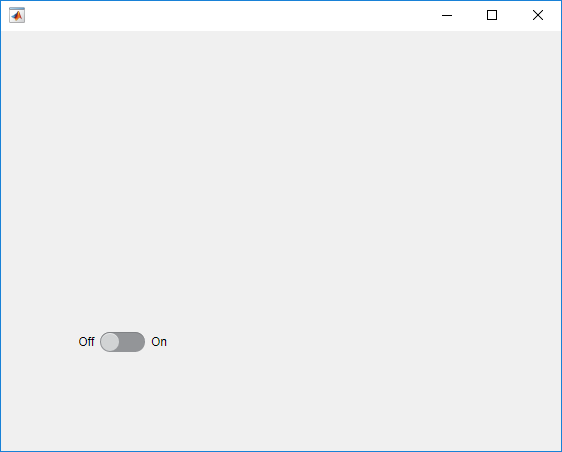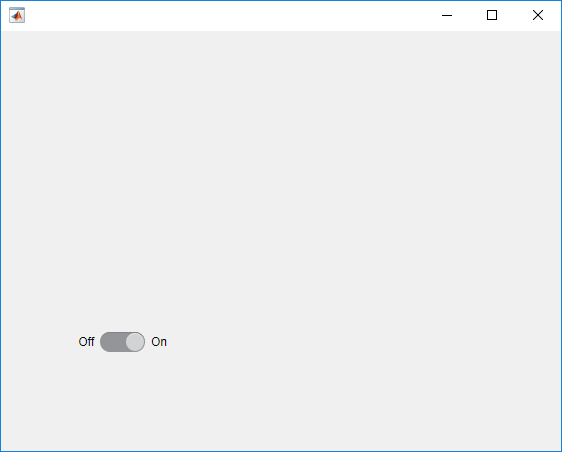# press

## 语法

``press(testcase,comp)``
``press(testcase,comp,location)``
``press(testcase,spn,direction)``
``press(testcase,compst,'SelectionType',type)``
``press(testcase,compst,location,'SelectionType',type)``

## 说明

` `press(testcase,comp)` 对 UI 组件 `comp` 执行按下手势。`

` `press(testcase,comp,location)` 指定组件内要按下的位置。您只能基于五个可用组件来指定位置：坐标区、UI 坐标区、极坐标区、面板和 UI 图窗。如果不指定位置，MATLAB® 会在 `comp` 的中心按下。`

` `press(testcase,spn,direction)` 指定是按下微调器 `spn` 中的 'up' 还是 'down' `direction` 按钮。`

` `press(testcase,compst,'SelectionType',type)` 使用指定的鼠标选择类型 `type` 对组件 `compst` 执行按下手势。`

` `press(testcase,compst,location,'SelectionType',type)` 使用指定的鼠标选择类型指定要在组件中按下的位置。`

## 输入参数

• 坐标区和 UI 坐标区 - 1×2 或 1×3 数值数组，其中包含 x、y 以及可选的 z 坐标。

• 极坐标区 - 1×2 数值数组，其中包含 θ 和 r 坐标。

• 面板和 UI 图窗 - 1×2 数值数组，其中包含 x 和 y 坐标。指定要按下的点的坐标，以组件左下角为起点测量（以像素为单位）。

UI 坐标区`uiaxes`
UI 图窗`uifigure`

`'normal'`

`'extend'`

• Shift-点击鼠标左键。

• 点击鼠标中键。

• 同时点击鼠标左键和右键。

`'alt'`

• Control-点击鼠标左键。

• 点击鼠标右键。

`'open'`

## 示例

`s = uiswitch('slider');````tc = matlab.uitest.TestCase.forInteractiveUse; tc.press(s);``````ax = uiaxes; tc = matlab.uitest.TestCase.forInteractiveUse; ```

`tc.press(ax)`

`tc.press(ax,[0.85 0.2])`

`b = uibutton('state');`

```tc = matlab.uitest.TestCase.forInteractiveUse; tc.verifyFalse(b.Value)```
`Verification passed.`

```tc.press(b) tc.verifyTrue(b.Value)```
`Verification passed.`

```s = uispinner('Value',42); initVal = s.Value;```

```tc = matlab.uitest.TestCase.forInteractiveUse; tc.press(s,'up') tc.verifyEqual(s.Value,initVal+s.Step)```
`Verification passed.`

```tc.press(s,'down') tc.verifyEqual(s.Value,initVal) ```
`Verification passed.`

```f = uifigure; tc = matlab.uitest.TestCase.forInteractiveUse; ```

`tc.press(f,'SelectionType','alt')`

`tc.press(f,[100 200],'SelectionType','open')`